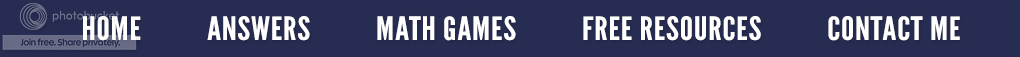### Using Free Milk Lid Jug Lids for Hands-On Math ActivitiesMilk Lid Math
Start saving milk jug lids because there are countless hands-on math activities you can do in your classroom using this free manipulative. Here are just four of those ideas.

1) Sort the lids by various attributes such as:
• Color
• Snap-on or Twist-on
• Label or No Label
• Kind of edge (smooth or rough)
2) Let the students grab one handful of lids.
• Ask the students to count the lids.
• See if the students can write that number.
3) Make a pattern using two different colors of lids.

• Identify the pattern using letters of the alphabet or numbers. The pattern above would be an A, A, B pattern or a 1, 1, 2 pattern.
• Now ask the students to use more than two colors to make a pattern
• Once more, have the students identify the pattern using alphabet letters or numbers.
4) Decide on a money value for each color of lid. (Example: Red lids are worth a nickel, blue lids are worth a dime, and white lids are worth a penny.) Put all of the lids into a bag and have the students draw out four lids. Have the students add up the total value of these four lids.
•\$3.00
Use play money (coins) to have the students show the value of the lids.
• Have the students practice writing money as either a part of a dollar or as cents.
• Another idea is to have the students find all the combinations of lids that would equal a nickel or a dime or a quarter.
The resource, Milk Lid Math, contains over 15 hands-on ideas with numerous activities listed under each one. These may be used with a whole group, small groups or as center activities.